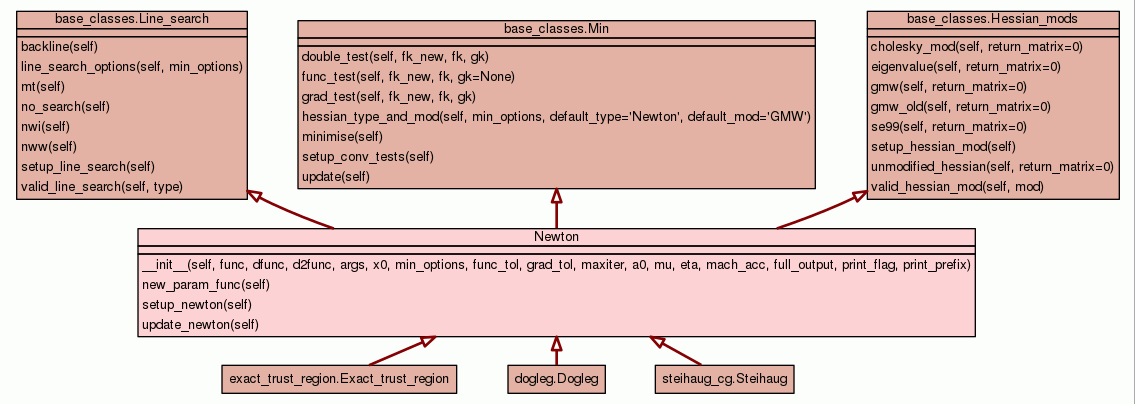[frames] | no frames]

# Class Newton

source codeInstance Methods

 __init__(self, func, dfunc, d2func, args, x0, min_options, func_tol, grad_tol, maxiter, a0, mu, eta, mach_acc, full_output, print_flag, print_prefix) Class for Newton minimisation specific functions. source code

 new_param_func(self) The new parameter function. source code

 setup_newton(self) Setup function. source code

 update_newton(self) Function to update the function value, gradient vector, and Hessian matrix. source code

Inherited from `base_classes.Hessian_mods`: `cholesky_mod`, `eigenvalue`, `gmw`, `gmw_old`, `se99`, `setup_hessian_mod`, `unmodified_hessian`, `valid_hessian_mod`

Inherited from `base_classes.Line_search`: `backline`, `line_search_options`, `mt`, `no_search`, `nwi`, `nww`, `setup_line_search`, `valid_line_search`

Inherited from `base_classes.Min`: `double_test`, `func_test`, `grad_test`, `hessian_type_and_mod`, `minimise`, `setup_conv_tests`, `update`

 Method Details

### __init__(self, func, dfunc, d2func, args, x0, min_options, func_tol, grad_tol, maxiter, a0, mu, eta, mach_acc, full_output, print_flag, print_prefix)(Constructor)

source code

Class for Newton minimisation specific functions.

Unless you know what you are doing, you should call the function 'newton' rather than using this class.

Overrides: base_classes.Min.__init__

### new_param_func(self)

source code

The new parameter function.

Find the search direction, do a line search, and get xk+1 and fk+1.

### setup_newton(self)

source code

Setup function.

The initial Newton function value, gradient vector, and Hessian matrix are calculated.

 Generated by Epydoc 3.0.1 on Fri Jun 14 11:29:44 2019 http://epydoc.sourceforge.net